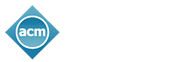## Estimating the Percolation Centrality of Large Networks through Pseudo-dimension Theory

Andre Vignatti: UFPR; Murilo da Silva: UFPR; Alane de Lima: UFPR

In this work we investigate the problem of estimating the percolation centrality of every vertex in a graph. This centrality measure quantifies the importance of each vertex in a graph going through a contagious process. It is an open problem whether the percolation centrality can be computed in O(n3-c) time, for any constant c>0. In this paper we present a ~O(m) randomized approximation algorithm for the percolation centrality for every vertex of G, generalizing techniques developed by Riondato, Upfal and Kornaropoulos. The estimation obtained by the algorithm is within ε of the exact value with probability 1- δ, for fixed constants 0 < ε,δ < 1. In fact, we show in our experimental analysis that in the case of real-world complex networks, the output produced by our algorithm is significantly closer to the exact values than its guarantee in terms of theoretical worst case analysis.

## How can we assist you?

We'll be updating the website as information becomes available. If you have a question that requires immediate attention, please feel free to contact us. Thank you!

Please enter the word you see in the image below: